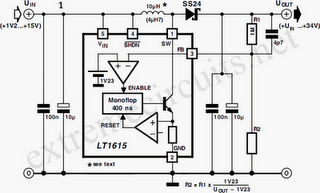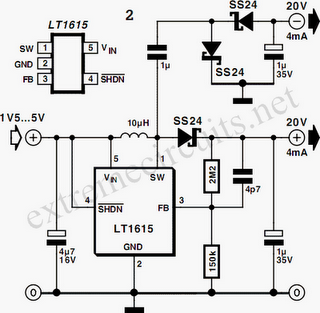# Learning Electronics

Learn to build electronic circuits

# DC/DC Converter From +1.5V To +34V

An interesting DC/DC converter IC is available from Linear Technology. The LT1615 step-up switching voltage regulator can generate an output voltage of up to +34V from a +1.2 to +15V supply, using only a few external components. The tiny 5-pin SOT23 package makes for very compact construction. This IC can for example be used to generate the high voltage needed for an LCD screen, the tuning voltage for a varicap diode and so on. The internal circuit diagram of the LT1615 is shown in Figure 1. It contains a monostable with a pulse time of 400 ns, which determines the off time of the transistor switch.

If the voltage sampled at the feedback input drops below the reference threshold level of 1.23 V, the transistor switches on and the current in the coil starts to increase. This builds up energy in the magnetic ﬁeld of the coil. When the current through the coil reaches 350 mA, the monostable is triggered and switches the transistor off for the following 400 ns. Since the energy stored in the coil must go somewhere, current continues to ﬂow through the coil, but it decreases linearly. This current charges the output capacitor via the Schottky diode (SS24, 40V/2A). As long as the voltage at FB remains higher than 1.23V, nothing else happens.As soon as it drops below this level, however, the whole cycle is repeated. The hysteresis at the FB input is 8mV. The output voltage can be calculated using the formula Vout = 1.23V (R1+R2) / R2 The value of R1 can be selected in the megohm range, since the current into the FB input is only a few tens of nano-amperes. When the supply voltage is switched on, or if the output is short-circuited, the IC enters the power-up mode. As long as the voltage at FB is less than 0.6V, the LT1615 output current is limited to 250mA instead of 350mA, and the monostable time is increased to 1.5µs.

These measures reduce the power dissipation in the coil and diode while the output voltage is rising. In order to minimize the noise voltages produced when the coil is switched, the IC must be properly decoupled by capacitors at the input and output. The series resistance of these capacitors should be as low as possible, so that they can short noise voltages to earth. They should be located as close to the IC as possible, and connected directly to the earth plane. The area of the track at the switch output (SW) should be as small as possible. Connecting a 4.7-µF capacitor across the upper feedback capacitor helps to reduce the level of the output ripple voltage.The selection of the coil inductance is described in detail in the LT1615 data sheet at www.linear-tech.com. Normally, a 4.7µH ﬁlter choke is satisfactory for output voltages less than 7V. For higher voltages, a 10-µH choke should be used. In the data sheet, the Coilcraft DO1608-472 (4.7 µF) and DO1608-100 (10 µF) are recommended. The Schottky diode must naturally have a reverse blocking voltage that is significantly greater than the value of the output voltage. The types MBR0530 and SS24 are recommended. The shutdown input (/SHDN) can be used to disable the step-up regulator by applying a voltage that is less than +0.25V.

If the voltage at this pin is +0.9 V or higher, the LT1615 is active. You must bear in mind that even when the IC is disabled, the input voltage still can reach the output via the coil and the diode, reduced only by the forward voltage drop of the diode. The second circuit diagram for the LT1615 (Figure 2) shows how you can make a symmetric power supply using this switching regulator. Here the switch output of the IC is tapped off and rectified using a symmetrical rectifier. The voltage divider at the positive output of the rectifier determines the output voltage.